# Slide 4 reformatted Slide 8 fixed grammar Slide

• Slides: 43Slide 4: reformatted Slide 8: fixed grammar Slide 14: fixed triple bonds Slide 16: added Robin Slide 18: reformatted Slide 23: I added the units to the Nutritional Guidelines. I was confused regarding what those numbers were in absence of the guidelines. What do you then skip from IS 6 to IS 8? ? ?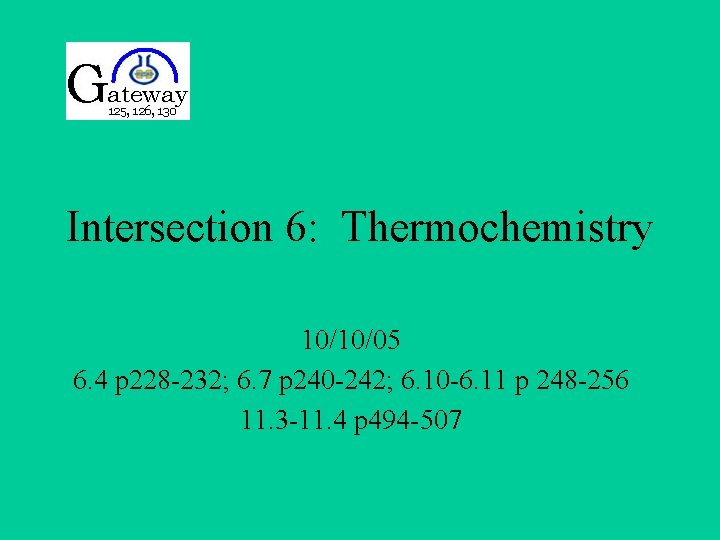Intersection 6: Thermochemistry 10/10/05 6. 4 p 228 -232; 6. 7 p 240 -242; 6. 10 -6. 11 p 248 -256 11. 3 -11. 4 p 494 -507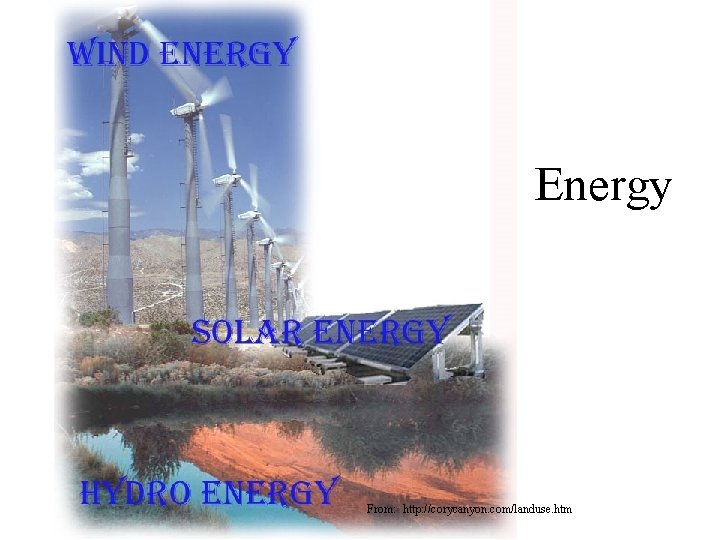Energy From: http: //corycanyon. com/landuse. htm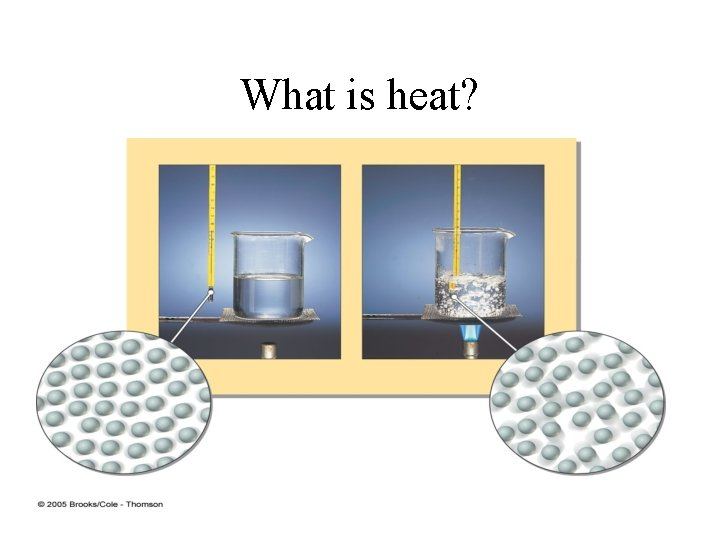What is heat?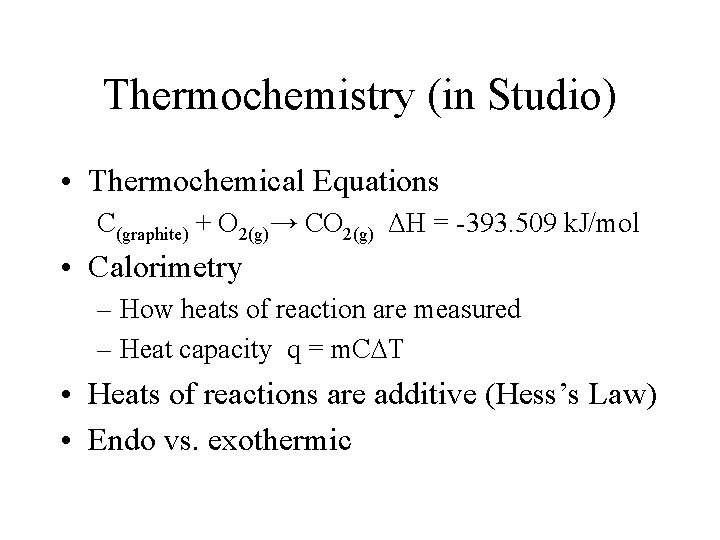Thermochemistry (in Studio) • Thermochemical Equations C(graphite) + O 2(g)→ CO 2(g) ΔH = -393. 509 k. J/mol • Calorimetry – How heats of reaction are measured – Heat capacity q = m. C T • Heats of reactions are additive (Hess’s Law) • Endo vs. exothermic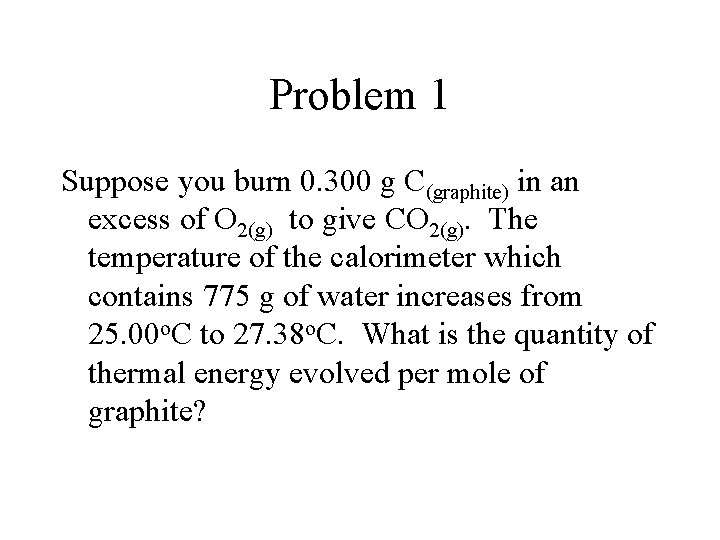Problem 1 Suppose you burn 0. 300 g C(graphite) in an excess of O 2(g) to give CO 2(g). The temperature of the calorimeter which contains 775 g of water increases from 25. 00 o. C to 27. 38 o. C. What is the quantity of thermal energy evolved per mole of graphite?Question 1 100 m. L of water at 25°C and 100 m. L of alcohol at 25°C are both heated at the same rate under identical conditions. After 3 minutes the temperature of the alcohol is 50°C. Two minutes later the temperature of the water is 50°C. Which liquid received more heat as it warmed to 50°C? a. The water. b. The alcohol. c. Both received the same amount of heat. d. It is impossible to tell from the information given. How do you know that?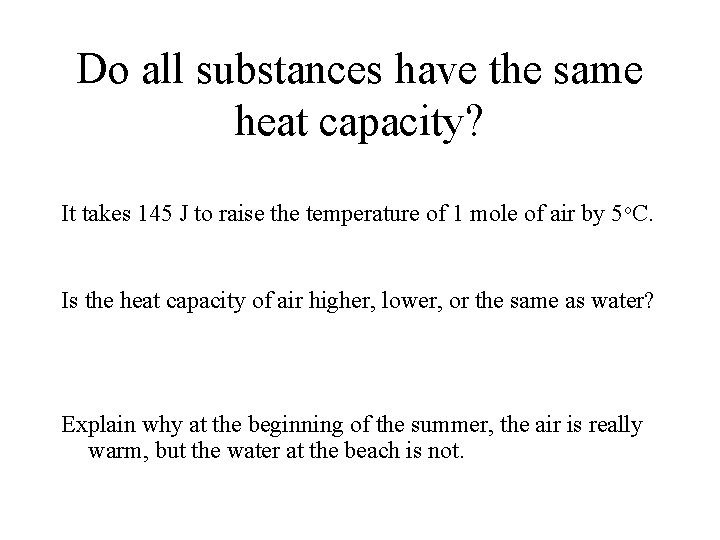Do all substances have the same heat capacity? It takes 145 J to raise the temperature of 1 mole of air by 5 o. C. Is the heat capacity of air higher, lower, or the same as water? Explain why at the beginning of the summer, the air is really warm, but the water at the beach is not.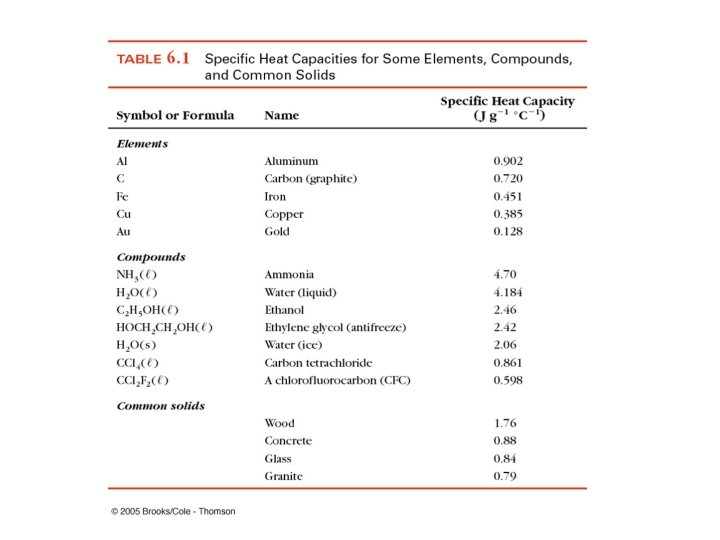Thermochemistry (in IS) • Heat and bonds – Break bonds takes energy – Make bonds get energy – Trends in bond enthalpy • Calculate Heats of Reactions – Heats of reaction can be calculated based on bond enthalpy – Heat of reactions can be calculated from heats of formation • Change of state – Solid to liquid – Liquid to gas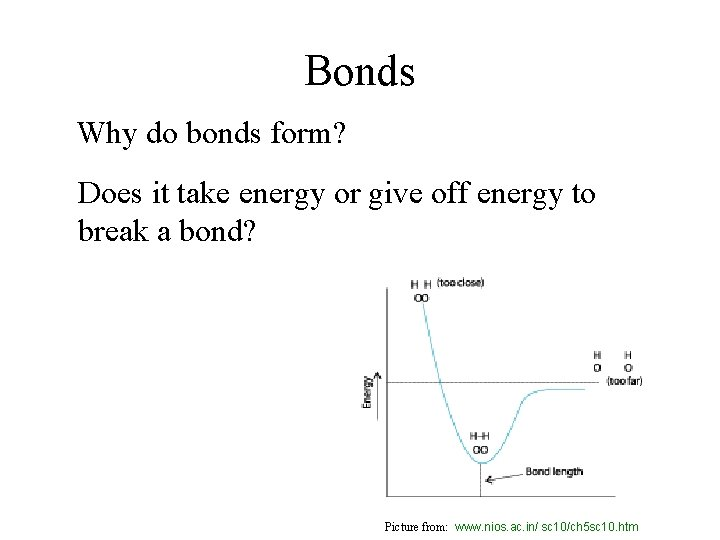Bonds Why do bonds form? Does it take energy or give off energy to break a bond? Picture from: www. nios. ac. in/ sc 10/ch 5 sc 10. htm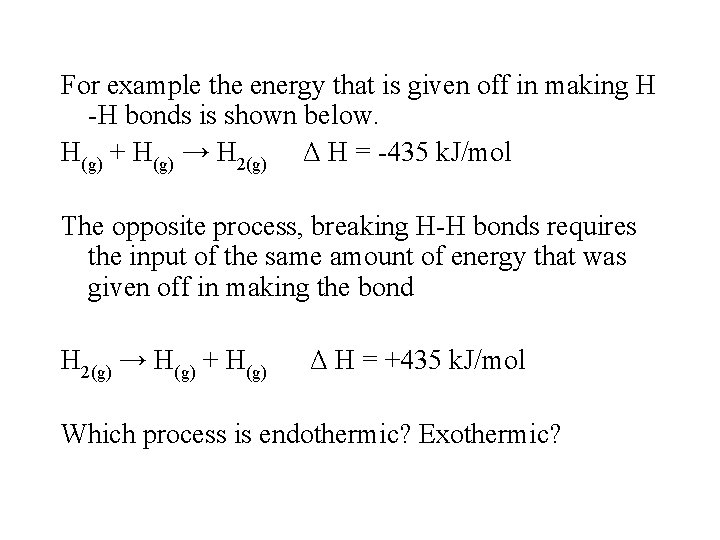For example the energy that is given off in making H -H bonds is shown below. H(g) + H(g) → H 2(g) Δ H = -435 k. J/mol The opposite process, breaking H-H bonds requires the input of the same amount of energy that was given off in making the bond H 2(g) → H(g) + H(g) Δ H = +435 k. J/mol Which process is endothermic? Exothermic?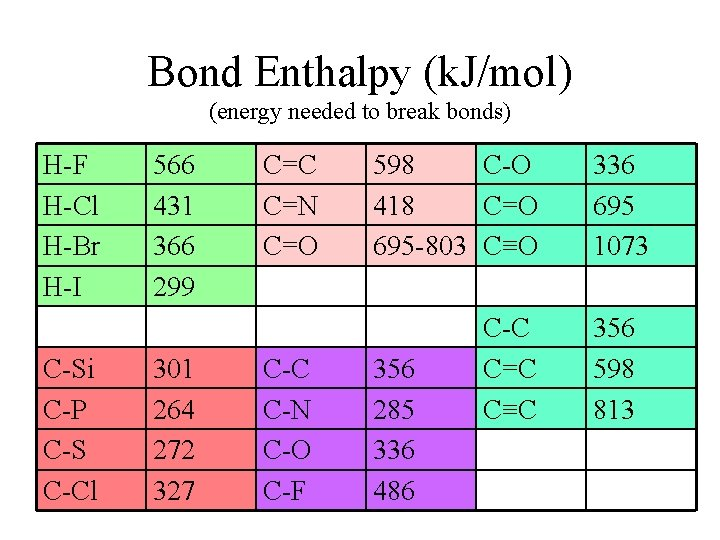Bond Enthalpy (k. J/mol) (energy needed to break bonds) H-F H-Cl H-Br H-I C-Si C-P C-S C-Cl 566 431 366 299 301 264 272 327 C=C C=N C=O C-C C-N C-O C-F 598 C-O 418 C=O 695 -803 C≡O 356 285 336 486 C-C C=C C≡C 336 695 1073 356 598 813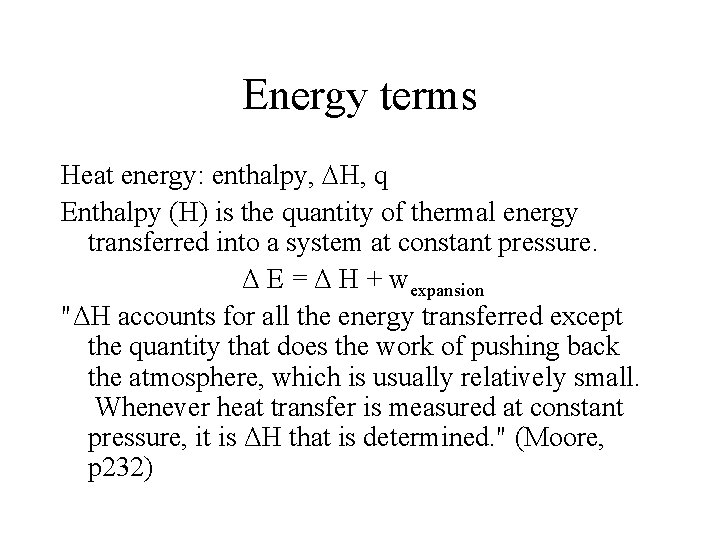Energy terms Heat energy: enthalpy, H, q Enthalpy (H) is the quantity of thermal energy transferred into a system at constant pressure. Δ E = Δ H + wexpansion "ΔH accounts for all the energy transferred except the quantity that does the work of pushing back the atmosphere, which is usually relatively small. Whenever heat transfer is measured at constant pressure, it is ΔH that is determined. " (Moore, p 232)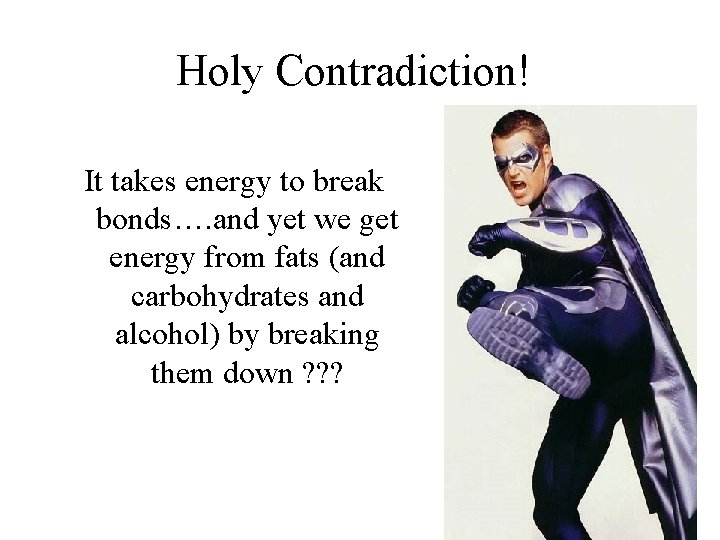Holy Contradiction! It takes energy to break bonds…. and yet we get energy from fats (and carbohydrates and alcohol) by breaking them down ? ? ?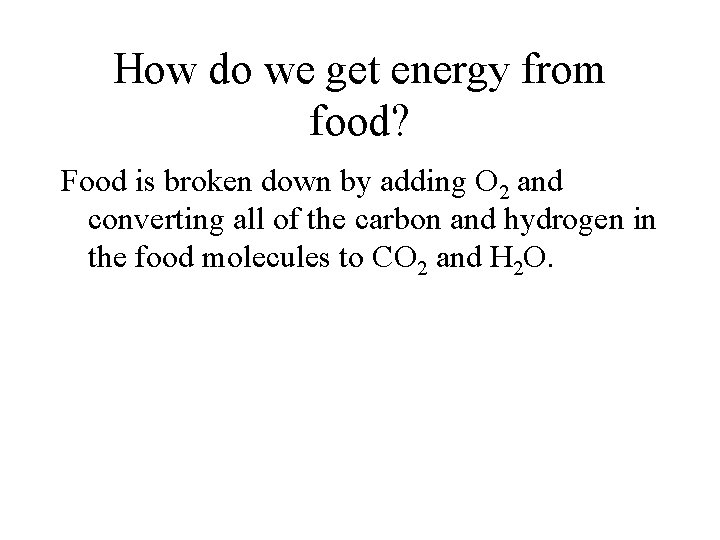How do we get energy from food? Food is broken down by adding O 2 and converting all of the carbon and hydrogen in the food molecules to CO 2 and H 2 O.Energy from Ethanol 3 2 → CO 2 3 CH 3 CH 2 OH + O 2 + H 2 O moles Bonds broken Enthalpies k. J/mol moles Bonds Enthalpies made k. J/mol 5 C-H 416 4 C=O 803 1 C-C 356 6 O-H 467 1 C-O 336 1 O-H 467 3 O=O 498 Total 4733 k. J (-) 6014 k. JA Picture of Breaking Down EthanolTotal H 4733 k. J into reactants 6014 k. J out of products Δ Hreaction = Σ Hreactants - Σ Hproducts = -1281 k. J Watch your signs!!!!!…. !!!!!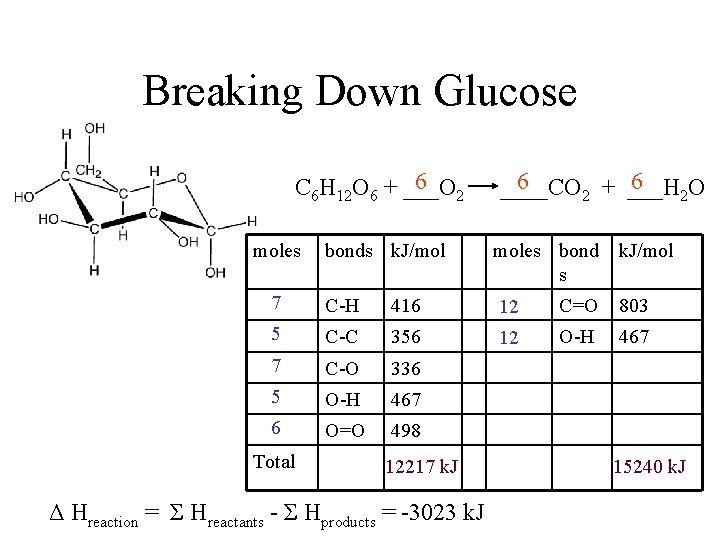Breaking Down Glucose 6 2 ____CO 6 6 C 6 H 12 O 6 + ___O 2 + ___H 2 O moles bonds k. J/mol moles bond s k. J/mol 7 C-H 416 12 C=O 803 5 C-C 356 12 O-H 467 7 C-O 336 5 O-H 467 6 O=O 498 Total 12217 k. J Δ Hreaction = Σ Hreactants - Σ Hproducts = -3023 k. J 15240 k. JConversion to food Calories • 1 Calorie = 1 kilocalorie = 1000 calories • 1 calorie = 4. 184 J • Convert the enthalpies for glucose and ethanol to Cal and Cal/g: For glucose 3023 k. J mol 1000 J 1 k. J 1 cal 4. 184 J 722. 5 Cal 1 mol glucose 180 g 1 Cal 1000 cal = 4. 0 Cal/g = 722. 5 Cal/mol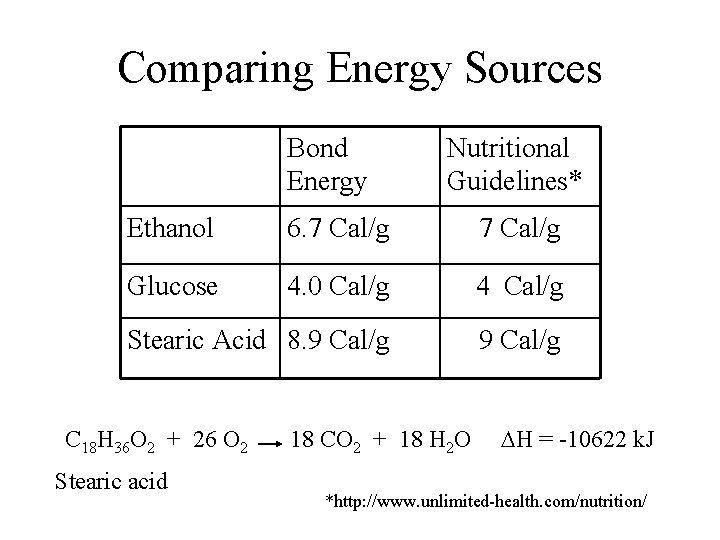Comparing Energy Sources Bond Energy Nutritional Guidelines* Ethanol 6. 7 Cal/g Glucose 4. 0 Cal/g 4 Cal/g Stearic Acid 8. 9 Cal/g C 18 H 36 O 2 + 26 O 2 18 CO 2 + 18 H 2 O H = -10622 k. J Stearic acid *http: //www. unlimited-health. com/nutrition/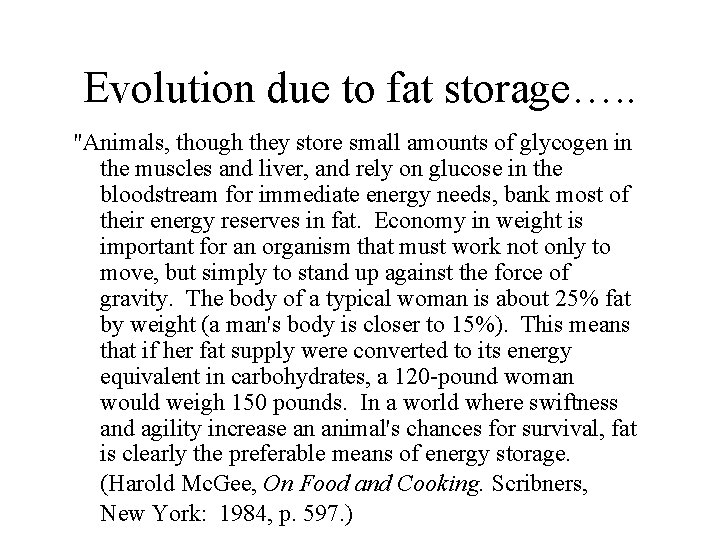Evolution due to fat storage…. . "Animals, though they store small amounts of glycogen in the muscles and liver, and rely on glucose in the bloodstream for immediate energy needs, bank most of their energy reserves in fat. Economy in weight is important for an organism that must work not only to move, but simply to stand up against the force of gravity. The body of a typical woman is about 25% fat by weight (a man's body is closer to 15%). This means that if her fat supply were converted to its energy equivalent in carbohydrates, a 120 -pound woman would weigh 150 pounds. In a world where swiftness and agility increase an animal's chances for survival, fat is clearly the preferable means of energy storage. (Harold Mc. Gee, On Food and Cooking. Scribners, New York: 1984, p. 597. )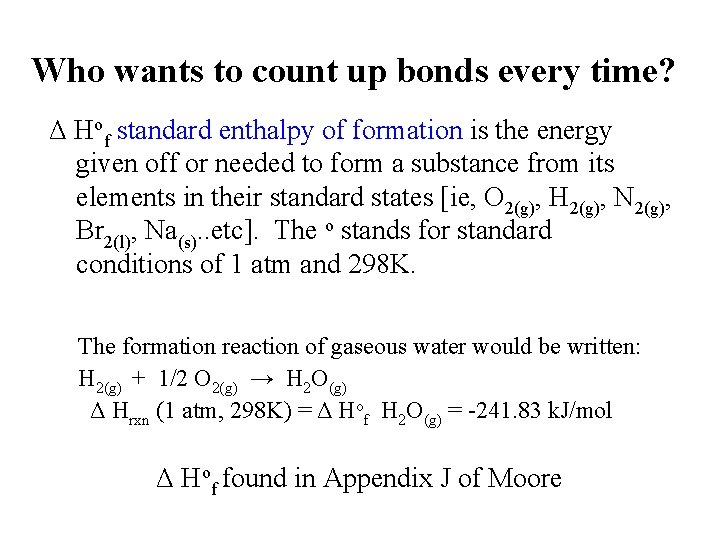Who wants to count up bonds every time? Δ Hof standard enthalpy of formation is the energy given off or needed to form a substance from its elements in their standard states [ie, O 2(g), H 2(g), N 2(g), Br 2(l), Na(s). . etc]. The o stands for standard conditions of 1 atm and 298 K. The formation reaction of gaseous water would be written: H 2(g) + 1/2 O 2(g) → H 2 O(g) Δ Hrxn (1 atm, 298 K) = Δ Hof H 2 O(g) = -241. 83 k. J/mol Δ Hof found in Appendix J of Moore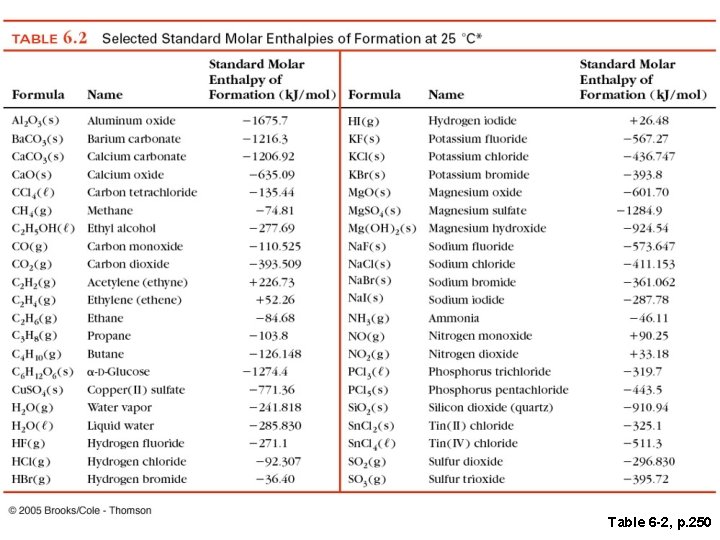Table 6 -2, p. 250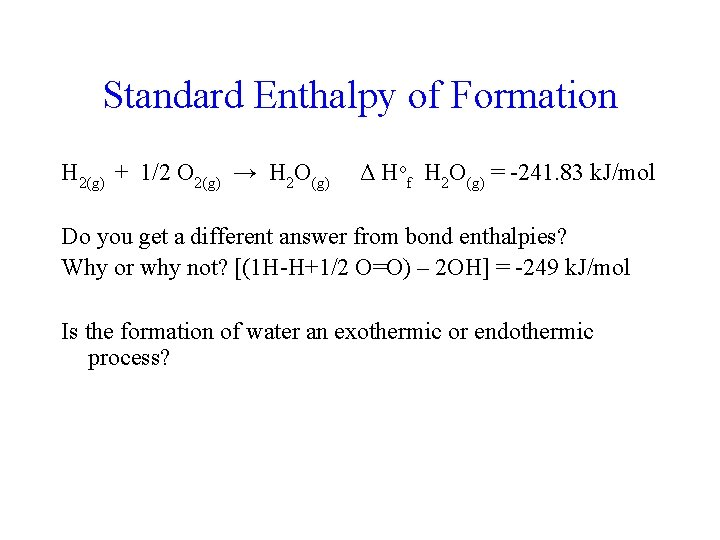Standard Enthalpy of Formation H 2(g) + 1/2 O 2(g) → H 2 O(g) Δ Hof H 2 O(g) = -241. 83 k. J/mol Do you get a different answer from bond enthalpies? Why or why not? [(1 H-H+1/2 O=O) – 2 OH] = -249 k. J/mol Is the formation of water an exothermic or endothermic process?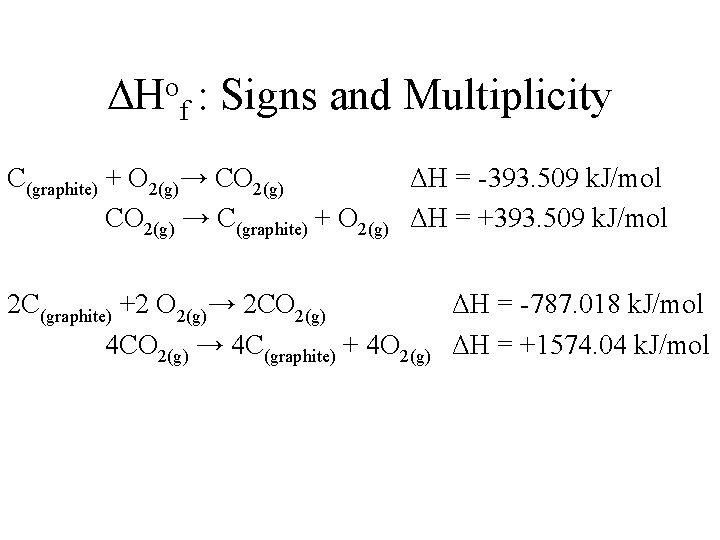o H f : Signs and Multiplicity C(graphite) + O 2(g)→ CO 2(g) ΔH = -393. 509 k. J/mol CO 2(g) → C(graphite) + O 2(g) ΔH = +393. 509 k. J/mol 2 C(graphite) +2 O 2(g)→ 2 CO 2(g) ΔH = -787. 018 k. J/mol 4 CO 2(g) → 4 C(graphite) + 4 O 2(g) ΔH = +1574. 04 k. J/mol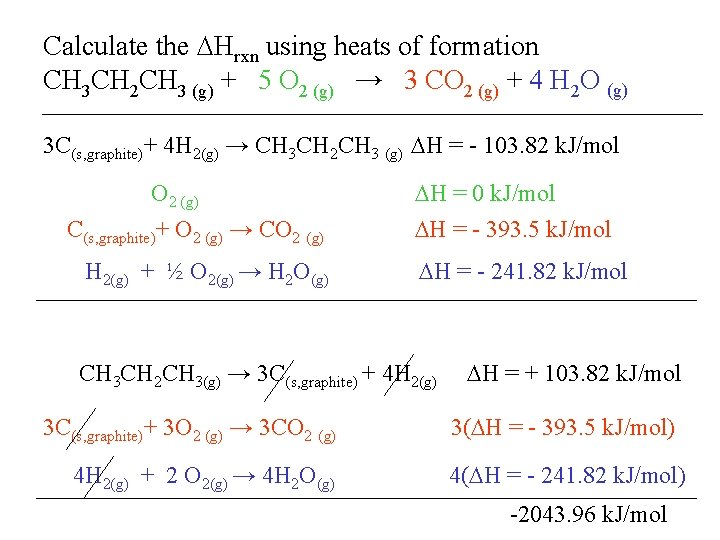Calculate the Hrxn using heats of formation CH 3 CH 2 CH 3 (g) + 5 O 2 (g) → 3 CO 2 (g) + 4 H 2 O (g) 3 C(s, graphite)+ 4 H 2(g) → CH 3 CH 2 CH 3 (g) H = - 103. 82 k. J/mol O 2 (g) H = 0 k. J/mol C(s, graphite)+ O 2 (g) → CO 2 (g) H = - 393. 5 k. J/mol H 2(g) + ½ O 2(g) → H 2 O(g) H = - 241. 82 k. J/mol CH 3 CH 2 CH 3(g) → 3 C(s, graphite) + 4 H 2(g) H = + 103. 82 k. J/mol 3 C(s, graphite)+ 3 O 2 (g) → 3 CO 2 (g) 3( H = - 393. 5 k. J/mol) 4 H 2(g) + 2 O 2(g) → 4 H 2 O(g) 4( H = - 241. 82 k. J/mol) -2043. 96 k. J/mol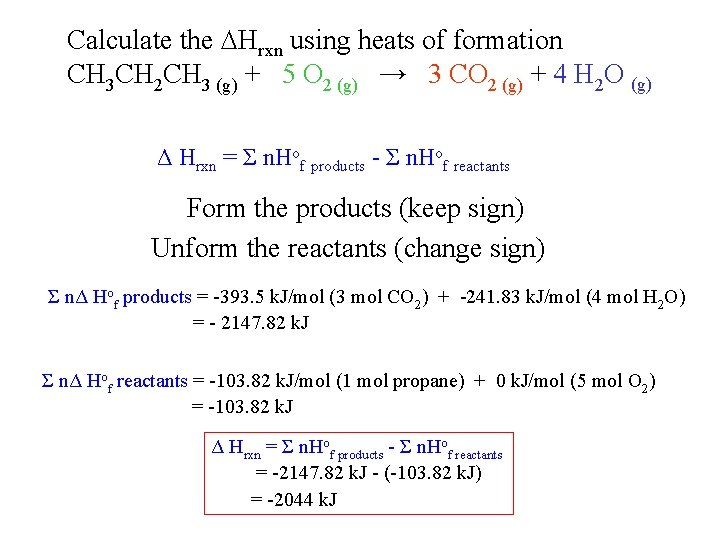Calculate the Hrxn using heats of formation CH 3 CH 2 CH 3 (g) + 5 O 2 (g) → 3 CO 2 (g) + 4 H 2 O (g) Δ Hrxn = Σ n. Hof products - Σ n. Hof reactants Form the products (keep sign) Unform the reactants (change sign) Σ nΔ Hof products = -393. 5 k. J/mol (3 mol CO 2) + -241. 83 k. J/mol (4 mol H 2 O) = - 2147. 82 k. J Σ nΔ Hof reactants = -103. 82 k. J/mol (1 mol propane) + 0 k. J/mol (5 mol O 2) = -103. 82 k. J Δ Hrxn = Σ n. Hof products - Σ n. Hof reactants = -2147. 82 k. J - (-103. 82 k. J) = -2044 k. J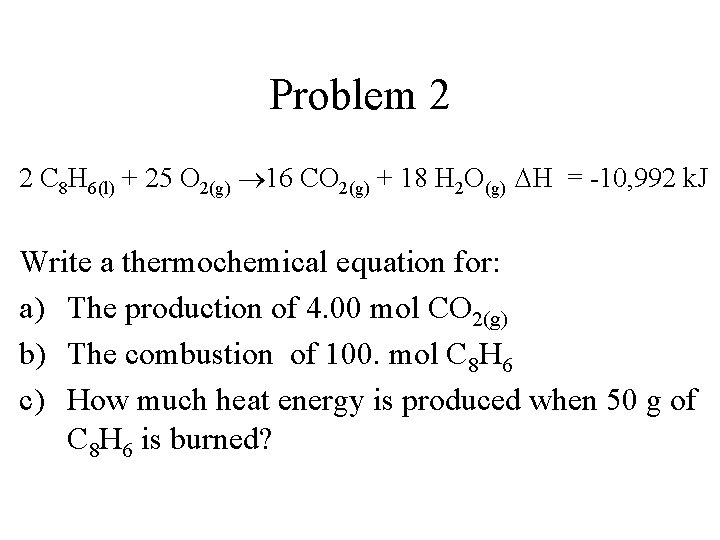Problem 2 2 C 8 H 6(l) + 25 O 2(g) 16 CO 2(g) + 18 H 2 O(g) H = -10, 992 k. J Write a thermochemical equation for: a) The production of 4. 00 mol CO 2(g) b) The combustion of 100. mol C 8 H 6 c) How much heat energy is produced when 50 g of C 8 H 6 is burned?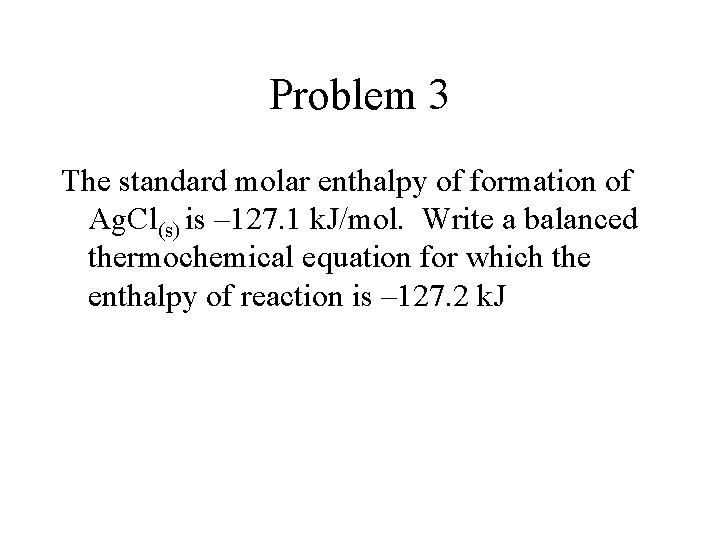Problem 3 The standard molar enthalpy of formation of Ag. Cl(s) is – 127. 1 k. J/mol. Write a balanced thermochemical equation for which the enthalpy of reaction is – 127. 2 k. J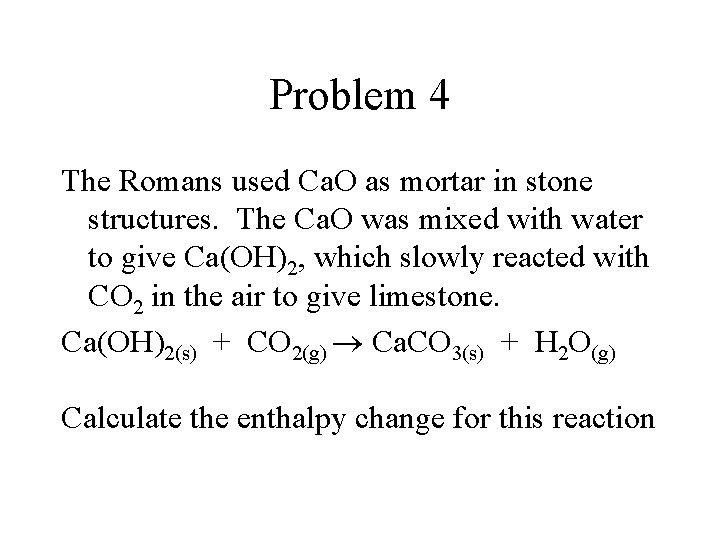Problem 4 The Romans used Ca. O as mortar in stone structures. The Ca. O was mixed with water to give Ca(OH)2, which slowly reacted with CO 2 in the air to give limestone. Ca(OH)2(s) + CO 2(g) Ca. CO 3(s) + H 2 O(g) Calculate the enthalpy change for this reaction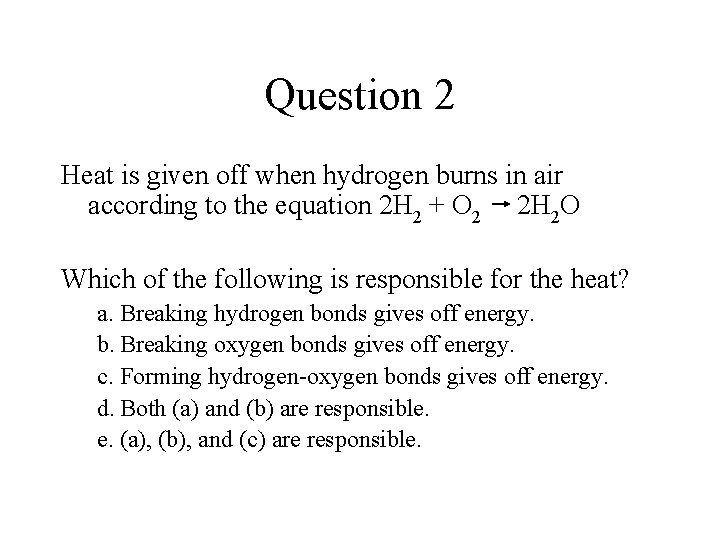Question 2 Heat is given off when hydrogen burns in air according to the equation 2 H 2 + O 2 2 H 2 O Which of the following is responsible for the heat? a. Breaking hydrogen bonds gives off energy. b. Breaking oxygen bonds gives off energy. c. Forming hydrogen-oxygen bonds gives off energy. d. Both (a) and (b) are responsible. e. (a), (b), and (c) are responsible.Heat and Phase Change • Why do we cool down when we sweat?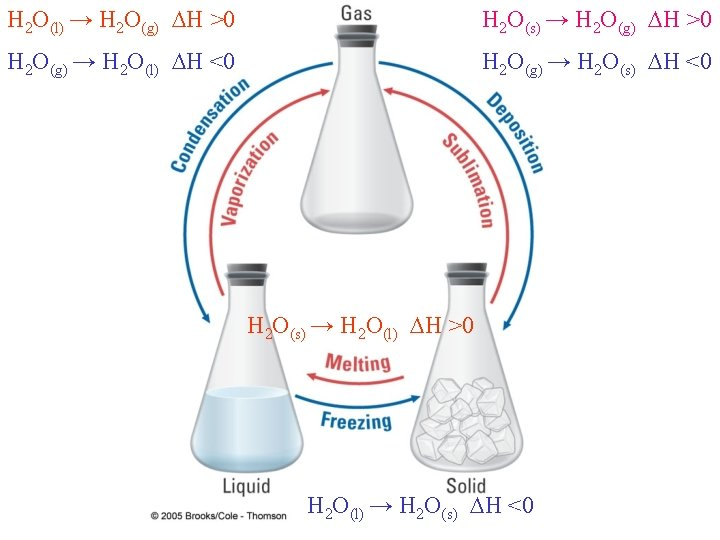H 2 O(l) → H 2 O(g) H >0 H 2 O(s) → H 2 O(g) H >0 H 2 O(g) → H 2 O(l) H <0 H 2 O(g) → H 2 O(s) H <0 H 2 O(s) → H 2 O(l) H >0 H 2 O(l) → H 2 O(s) H <0Enthalpies of Vaporization H 2 O(l) → H 2 O(g) H >0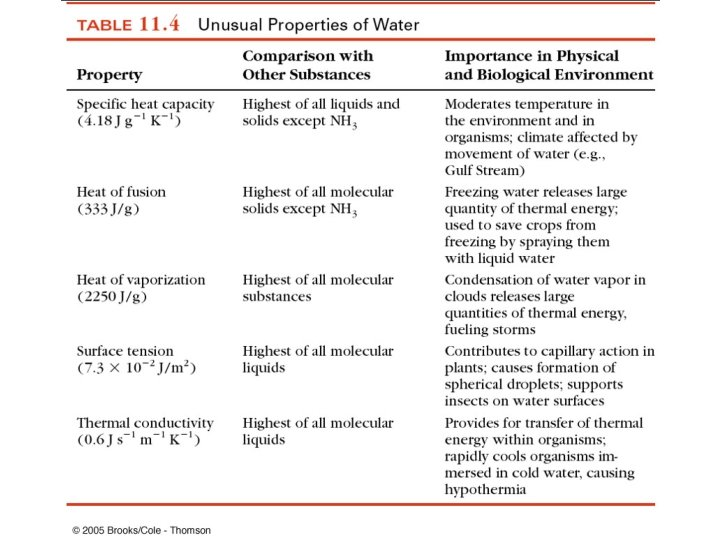Problem 5 Suppose that you add a small ice cube to room temperature (25 o. C) water in a coffee cup calorimeter. What is the final temperature when all of the ice is melted? Assume that you have 200. m. L of water and that the ice cube weighs 15. 0 g and is at 0 o. C before being added to the water. Heat of fusion of water = 333 J/gProblem 6 If you want to convert 56. 0 g ice (at 0 o. C) to water at 75. 0 o. C, how many grams of propane (C 3 H 8) would you have to run to supply the energy to melt the ice and then warm it to the final temperature?